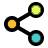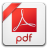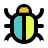Math Calculators, Lessons and Formulas

It is time to solve your math problem

mathportal.org

# Polynomial factoring calculator

This calculator is a free online math tool that writes a polynomial in factored form.
The solver shows a complete step-by-step explanation.

problem

Factor polynomial $x^{2}+20x+100$.

solution

$$x^{2}+20x+100 = ( x+10 )^{ 2 }$$

explanation

Both the first and third terms are perfect squares.

$$x^2 = \left( \color{blue}{ x } \right)^2 ~~ \text{and} ~~ 100 = \left( \color{red}{ 10 } \right)^2$$

The middle term ( $20x$ ) is two times the product of the terms that are squared.

$$20x = 2 \cdot \color{blue}{x} \cdot \color{red}{10}$$

We can conclude that the polynomial $x^{2}+20x+100$ is a perfect square trinomial, so we will use the formula below.

$$A^2 + 2AB + B^2 = (A + B)^2$$

In this example we have $\color{blue}{ A = x }$ and $\color{red}{ B = 10 }$ so,

$$x^{2}+20x+100 = ( \color{blue}{ x } + \color{red}{ 10 } )^2$$

## Report an Error !

Script name : polynomial-factoring-calculator

Form values: 100+20x+x^2 , g , factor 100+20x+x^2 - mathportal , ,

Comment (optional)

## Share Result

Or just copy and paste the link wherever you need it.Polynomial Factoring Calculator (shows all steps)
supports polynomials with both single and multiple variables
show help ↓↓ examples ↓↓ tutorial ↓↓
2x^2 + 3x - 5
10ab + 15b -2a - 3
9u^2v^4 + 6uv^2 + 1
working...
EXAMPLES
example 1:ex 1:
factor $x^2 - 10x + 25$
example 2:ex 2:
factor $3x^2 - 27$
example 3:ex 3:
factor $4x^3 - 21x^2 + 29x -6$
example 4:ex 4:
factor $3uv-12u+6v-24$
example 5:ex 5:
factor $a^2 + 2a + 1 - b^2$
Search our database of more than 200 calculators
TUTORIAL

## Polynomial Factoring Techniques

To find the factored form of a polynomial, this calculator employs the following methods:

1. Factoring GCF, 2 Factoring by grouping, 3 Using the difference of squares, and 4 Factoring Quadratic Polynomials

### Method 1 : Factoring GCF

Example 01: Factor $3ab^3 - 6a^2b$

\begin{aligned} 3ab^3 - 6a^2b &= \color{blue}{3 \cdot a \cdot b} \cdot b \cdot b - 2 \cdot \color{blue}{3 \cdot a } \cdot a \color{blue}{\cdot b} = \\[1 em] &= \color{blue}3ab(b^2-2a) \end{aligned}

### Method 2 : Factoring By Grouping

The method is very useful for finding the factored form of the four term polynomials.

Example 03: Factor $2a - 4b + a^2 - 2ab$

We usually group the first two and the last two terms.

$$2a - 4b + a^2 - 2ab = \color{blue}{2a - 4b} + \color{red}{a^2 - 2ab}$$

We now factor $\color{blue}{2}$ out of the blue terms and $\color{red}{a}$ out of from red ones.

$$\color{blue}{2a - 4b} + \color{red}{a^2 - 2ab} = \color{blue}{2 \cdot (a - 2b)} + \color{red}{a \cdot (a - 2b) }$$

At the end we factor out common factor of $(a - 2b)$

$$2 \cdot \color{blue}{(a - 2b)} + a \cdot \color{blue}{(a - 2b)} = (a - 2b) (2 + a)$$

Example 04: Factor $5ab + 2b + 5ac + 2c$

This is a rare situation where the first two terms of a polynomial do not have a common factor, so we have to group the first and third terms together.

\begin{aligned} 5ab + 2b + 5ac + 2c &= \color{blue}{5ab + 5ac} + \color{red}{2b + 2c} = \\ &=\color{blue}{5a (b + c)} + \color{red}{2 (b + c)} = \\ &= (b+c)(\color{blue}{5a} + \color{red}{2}) \end{aligned}

### Method 3 : Special Form - A

The most common special case is the difference of two squares

$$a^2 - b^2 = (a+b) (a-b)$$

We usually use this method when the polynomial has only two terms.

Example 05: Factor $4x^2 - y^2$.

First, we need to notice that the polynomial can be written as the difference of two perfect squares.

$$\color{blue}{4x^2} - \color{red}{y^2} = \color{blue}{ \left(2x\right)}^2 - \color{red}{y}^2$$

Now we can apply above formula with $\color{blue}{a = 2x}$ and $\color{red}{b = y}$

$$\left( \color{blue}{2x} \right)^2 - \color{red}{y}^2 = (2x - b) (2x + b)$$

Example 06: Factor $9a^2b^4 - 4c^2$.

The binomial we have here is the difference of two perfect squares, thus the calculation will be similar to the last one.

$$9a^2b^4 - 4c^2 = \left( 3ab^2 \right)^2 - \left( 2c \right)^2 = (3ab^2-2c)(3ab^2+2c)$$

### Method 4 : Special Form - B

The second special case of factoring is the Perfect Square Trinomial

$$a^2 \pm 2ab + b^2 = (a \pm b)^2$$

Example 07: Factor $100 + 20x + x^2$

In this case, the first and third terms are perfect squares. So we can use the above formula.

$$\color{blue}{100} + 20x + \color{red}{x^2} = \color{blue}{10}^2 + 2 \cdot \color{blue}{10} \cdot \color{red}{x} + \color{red}{x}^2 = (10 + x)^2$$

### Method 5: Factoring Quadratic Polynomials

The best way to explain this method is by using an example.

Example 08: Factor $x^2 + 3x + 4$

We know that the factored form has the following pattern

$$x^2 + 5x + 4 = (x + \_ ) (x + \_ )$$

All we have to do now is fill in the blanks with the two numbers. To do this, notice that the product of these two numbers has to be 4 and their sum has to be 5. We conclude, after some trial and error, that the missing numbers are $\color{blue}{1}$ and $\color{red}{4}$. As a result, the solution is::

$$x^2 + 5x + 4 = (x + \color{blue}{1} ) (x + \color{red}{4} )$$

Example 09: Factor $x^2 - 8x + 15$

Like in the previous example, we look again for the solution in the form

$$x^2 - 8x + 15 = (x + \_ ) (x + \_ )$$

The sum of missing numbers is $-8$ so we need to find two negative numbers such that the product is $15$ and the sum is $-8$. It's not hard to see that two numbers with such properties are $-3$ and $-5$, so the solution is.

$$x^2 + 5x + 4 = (x + \color{blue}{-3} ) (x + \color{red}{-5} ) = (x-3)(x-5)$$
Search our database of more than 200 calculators